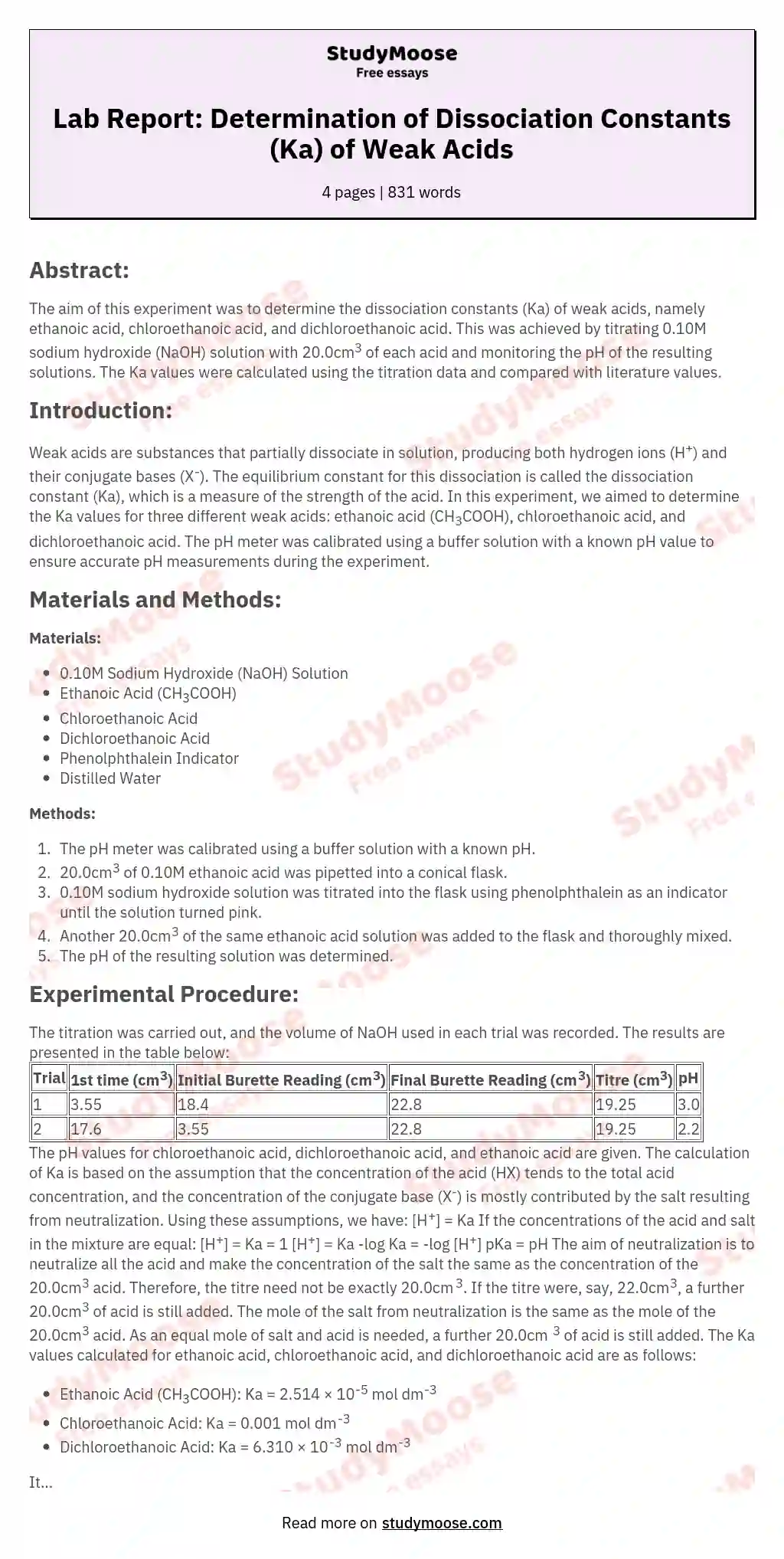# To find out the Ka of ethanoic acid, chloroethanoic acid and dichloroethanoic acid

1. The pH meter is calibrated, using a buffer solution of accurately known pH.

2. 20.0cm3 of 0.10M ethanoic acid was pipetted into a conical flask.

3. 0.10M sodium hydroxide solution was titrated using phenolphthalein as indicator, until the solution was just turned pink.

4. A further 20.0cm3 of the same ethanoic acid solution was added to the flask and was mixed thoroughly.

5. The pH of the resulting solution was determined.

Result:

Volume of NaOH used is recorded below:

Trial (cm3)

1st time (cm3)

3.55

18.4

22.8

36

Titre

19.25

17.6

pH

Chloroethanoic acid

3.0

Dichloroethanoic acid

2.2

Ethanoic acid

4.6

pH of chloroethanoic acid and dichloroethanoic acid is given by the teacher.

Calculation:

Ka =

1. It is reasonable to make the following assumptions:

[HX] ??total acid concentration

[X-] ??total salt concentration

because CH3COOH is a weak acid which is only slightly ionized and thus contributed only a little bit to total salt concentration. Therefore [HX] tends to total acid concentration, and [X-] is mostly contributed by the salt resulting from neutralization.

Get quality help nowProf. FinchVerified writer

Proficient in: Concentration4.7 (346)

“ This writer never make an mistake for me always deliver long before due date. Am telling you man this writer is absolutely the best. ”+84 relevant experts are online

Using these assumptions, we have:

[H+] ? Ka

2. If the concentrations of the acid and salt in the mixture are equal, then:

[H+] ? Ka ? 1

[H+] ? Ka

3. [H+] ? Ka

-log Ka = -log [H+]

pKa = pH

4. The aim of neutralization is to neutralize all the acid, and make the concentration of the salt same as the concentration of the 20.0cm3 acid. Therefore the titre need not be exactly 20.0cm3.

5. If the titre were, say 22.0cm3, a further 20.0cm3 of acid is still added. The mole of the salt from neutralization is same as the mole of 20.

Get to Know The Price Estimate For Your Paper
Topic
Number of pages
Email Invalid email

You won’t be charged yet!

0cm3 acid. As equal mole of salt and acid is needed, a further 20.0cm3 of acid is still added.

6. For CH3COOH,

pKa = pH

pKa = 4.6

Ka = 2.514 ? 10-5 mol dm-3

For Chloroethanoic acid,

pKa = 3.0

Ka = 0.001 mol dm-3

For Dichloroethanoic acid

pKa = 2.2

Ka = 6.310 ? 10-3 mol dm-3

Compare with literature value, this value show the greatest divergence from the accepted values

7. This is because assumption is made in my calculation. The slightly ionized acid contributes to the divergence. Consider the following equation:

Ka =

As some acid dissociated to [X-], [X-] is greater than we assumed and [HX] is smaller than we assumed. Thus Ka found from this experiment is greater than the accepted value.

8. Some suggestions are made to explain the relative values of Ka for the three acids. The more the chlorine atom an acid has, the greater the dissociation percentage for this acid. The greater the dissociation percentage for this acid, the greater is the [X-] and the smaller is the [HX], and then the Ka is also greater.

9. This method can also be used to determine the dissociation constant of weak bases. For ammonia:

Kb =

[OH-] ? Kb

Procedure 1~5 can be repeated while the acid is replaced by NH3 and NaOH is replaced by HCl. For the pH is known, by

14 – pH = pKb

the Kb can be found out.

Conclusion:

Ka

ethanoic acid

2.514 ? 10-5

chloroethanoic acid

0.001

dichloroethanoic acid

6.310 ? 10-3

Discussion:

1. Distilled water can be added to buffer solution. But Distilled water can not be added to the conical flask because it will alter the pH of the solution

2. The pH meter should be calibrated by washing it with deionized water and then with the acid.